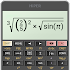# HiPER Scientific Calculator v10.0.5

Free

### HiPER Scientific Calculator / Screenshots### HiPER Scientific Calculator / Description

HiPER Scientific Calculator is a popular calculator with more than 25 million downloads and 100 000 five-star ratings.

The calculator has up to 100 digits of significand and 9 digits of exponent. It detects repeating decimals and numbers can be also entered as fractions or converted to fractions.

You can write expressions in a natural way and watch your calculations. The result is displayed as a number simplified expression etc.

The calculator has several layouts suitable for various screen sizes:
- "pocket" for small devices
- "compact" for smartphones (in portrait and landscape orientation)
- "expanded" for tablets

A multiline display can be turned on in tablets to show the complete history of calculations and to provide access the previous results.

Users can choose from several high-quality themes.

The calculator has many functions such as:
- basic arithmetic operations including percentage modulo and negation;
- fractions (in the expression mode any expression including nested fractions can be entered as a numerator and a denominator);
- mixed numbers;
- periodic numbers and their conversion to fractions;
- unlimited number of braces;
- operator priority;
- repeated operations;
- equations
- variables and symbolic computation;
- derivatives and integrals;
- graphs of functions and integral area 3D graphs;
- calculation details - extended information about a calculation like all complex roots unit circle etc.;
- complex numbers
- conversion between rectangular and polar coordinates
- sums and products of sequences
- advanced number operations such as random numbers combinations permutations common greatest divisor etc.;
- trigonometric and hyperbolic functions;
- powers roots logarithms etc.;
- degrees minutes and seconds conversion;
- fixed point scientific and engineering display format;
- display exponent as SI units prefix;
- memory operations with 10 extended memories;
- clipboard operations with various clipboard formats;
- result history;
- binary octal and hexadecimal numeral systems;
- logical operations;
- bitwise shifts and rotations;
- haptic feedback;
- more than 90 physical constants;
- conversion among 250 units;
- Reverse Polish notation.

The calculator has many settings to manage the full screen mode decimal and thousand separators etc.

All features are described with a built-in help.

### HiPER Scientific Calculator / What's New in v10.0.5

- Arithmetics with infinities
- Sum and product of infinite series
- Definite integral with infinite limits
- Limit
- New detail: Other types of limit
- New setting: Infinite results of finite expressions
- New functions: cotangent, secant, cosecant
- New function: decimal part
- Rounding functions accept matrix in parameter
- Improved factorial and Gamma function calculations
- New menus in custom keyboards
- New language: Ukrainian